# Lecture 13: Binary Star Systems, Masses of Stars

Chapter 17: pages 442 - 456

## How to find the masses of stars ?

Well, you weigh them, i.e measure gravitational interaction with something else.

## Binary Stars

• Most stars are not isolated in space.
• About one half of all stars which we see are in a double or multiple star system.
• In a multiple star system, the stars all orbit about the common centre-of-mass of the system.
• This is not accidental! The process of star formation tends to cause many stars to be formed close to each other.
 Hubble Space Telescope photo of Gliese 623, two stars separated by 2 AU.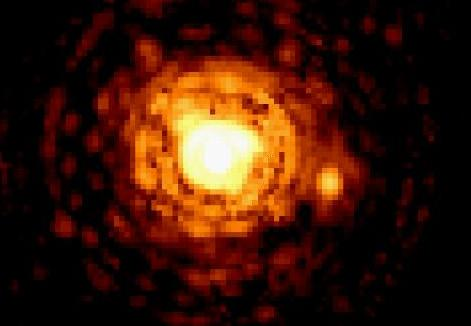Back Next

## Centre Of Mass (COM)

 Massive objects orbit around Center of Mass Suppose the two stars have masses M1 and M2. Let r1 = distance between star 1 and COM. Let r2 = distance between star 2 and COM. The Centre-Of-Mass Formula is r1M1 = r2M2.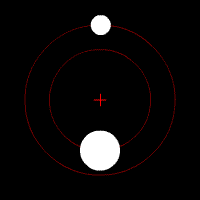The centre of mass is always closer to the heavier star. This is just like two kids playing on a teeter-totter. The heavier child must sit closer to the pivot point than the lighter child.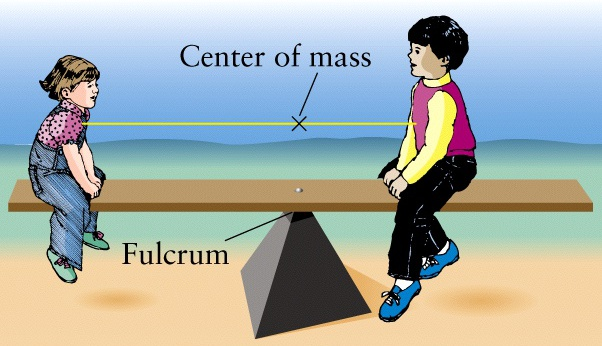• COM is invisible, but its position if the total distance between the two stars r = r1 + r2 has been measured is r1 = r M2/(M1+M2)
• A special case is for circular orbits. Each star is always at the same distance (equal the radii a1 and a2 of the orbits) from COM, so
a1M1 = a2M2.
Back Next

## An Ellipse

• In all binary systems both stars move in elliptical orbits about the centre-of-mass (COM).
• The semi-major axis is one-half the long axis of the ellipse.
• A circle is a special case of an ellipse with semi-major axis equal to the circle's radius.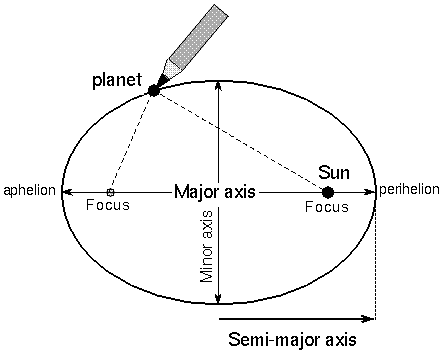Back Next

## Elliptical Orbits

• In a binary system, each star moves on an elliptical path.
• The COM sits at the focus for both ellipses.
• The distances between each star and the COM change with time, but r1M1 = r2M2.
• At all times, the stars are on opposite sides of the COM, so they never collide.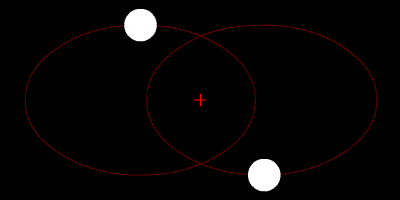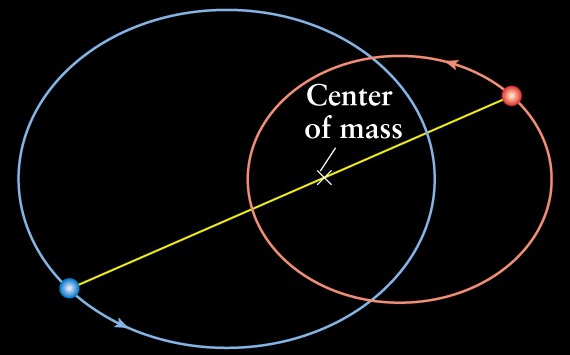The orbit of star 1 has semimajor axis a1. The orbit of star 2 has semimajor axis a2. The semimajor axes obey the same COM formula: a1M1 = a2M2. We can still define a = a1 + a2. What about elliptical orbit around the Sun? If you consider one star at rest (put an observer on this star !), it will appear to be at the focus of a larger ellipse with semimajor axis a that the other star moves along !
Back Next

## An Optical Double

• This is not a true binary star system, just a chance alignment of two stars in the same line-of-sight.
• An example of an optical double are the stars Alcor and Mizar which appear to be in the same location of the sky at the location marked "zeta" in the Big Dipper.
• If your eyesight is very good and the sky is dark, you can see that at the location of Mizar there are actually two stars, Alcor and Mizar.
• The distance between Alcor and Mizar is about 1 parsec, similar to the distance between the Sun and Alpha Centauri (our nearest stellar neighbour).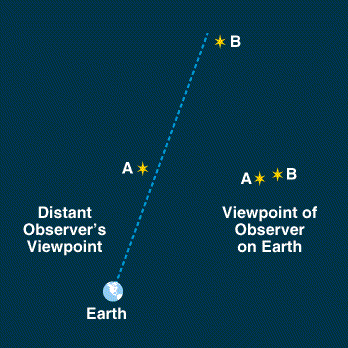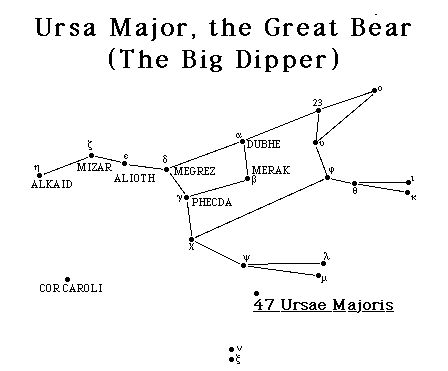Back Next

## A Visual Binary

• A double star system where you can see both stars and they appear to move around each other is a visual binary.
• An example is the star system Kruegar 60.
• The photos below show that from 1908 to 1920 the visual binary completed about 1/4 of a revolution.1908 1915 1920
 Another example of a Visual Binary is 70 Ophiuchi. The figure shows a plot of one star with respect to the other over time. Over the course of 87.7 years, the star makes one full orbit.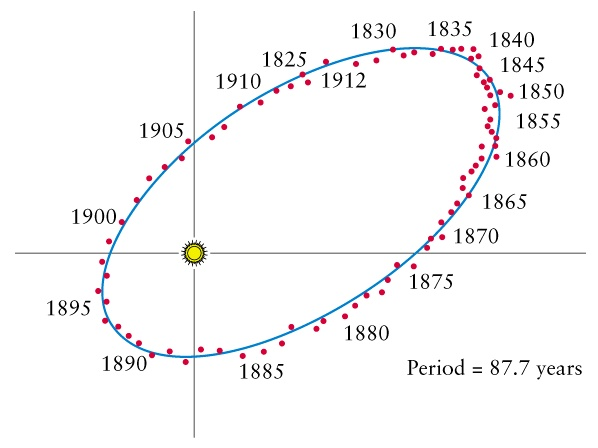Back Next

## Astrometric Binary

• If only one star is visible, but we can detect that it wobbles about an unseen centre-of-mass, then we have an astrometric binary.
• The star Sirius is actually a binary system, but Sirius A is so much brighter than Sirius B that we only see Sirius A.
• Sirius B was first detected by observing the motion of the brighter Sirius A.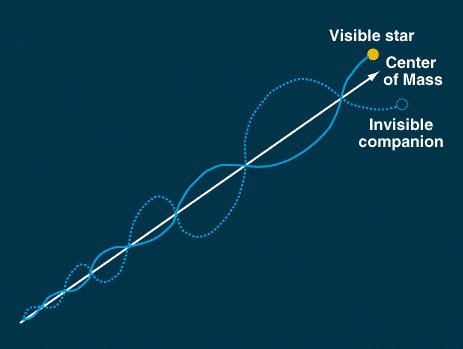Back Next

## Spectroscopic Binary

• We see only one source of light.
• The spectrum of the star shows Doppler shifts which change from redshift to blueshift periodically.
• This means that the star is moving in a periodic orbit.
• When the star moves towards us, we see a blueshift.
• When the star moves away from us, we see a redshift.
• The period of time from redshift to blueshift back to redshift again is the orbital period.
• Examples of spectroscopic binaries:
• Mizar A is actually a spectroscopic binary composed of two stars.
• Mizar B is also a spectroscopic binary composed of two stars.
• Mizar is actually a 4-star system.

Back Next

 Star A's light is blueshifted. Star B's light is redshifted.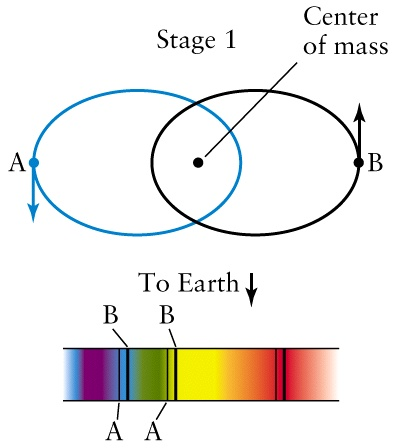No Doppler shifts occur.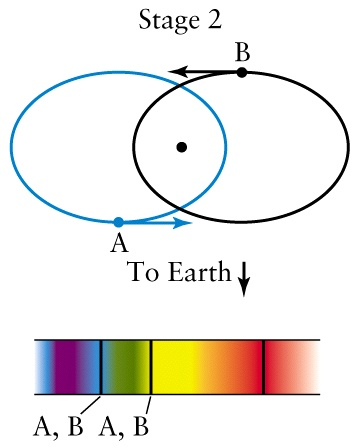Star A's light is redshifted. Star B's light is blueshifted.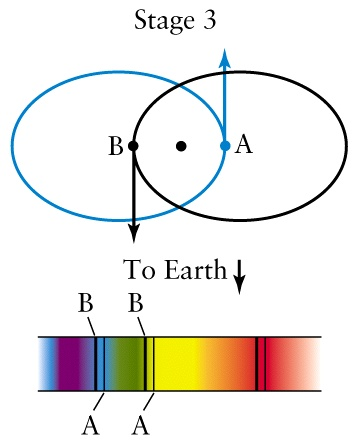No Doppler shifts occur.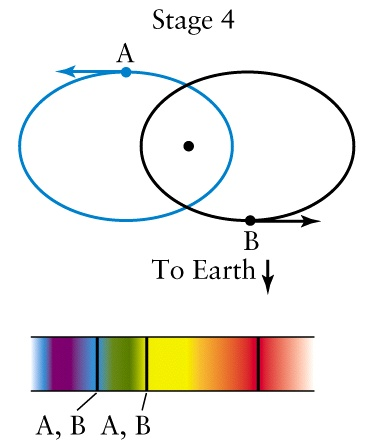Back Next

## Stellar Velocities in a Spectroscopic Binary

• From the Doppler shift data, we can reconstruct the component of the stars' velocities in our line of sight.
• The true velocities are only known if the binary's inclination angle with our line of sight is known.
• A typical velocity curve is shown.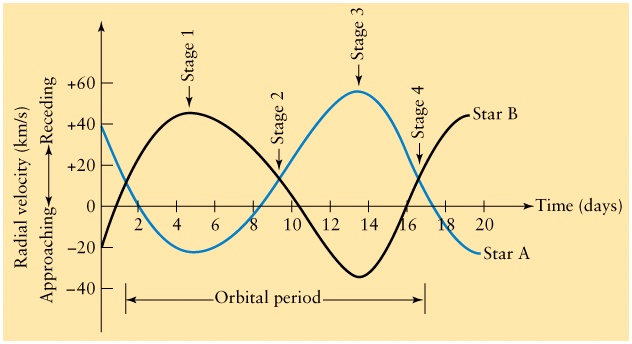Figure 19-24
Back Next

## Extra-Solar Planets

• This method is used to find planets around other stars.
• When a star has a planet, the system's centre-of-mass is not exactly at the centre of the star.
• (For example the centre-of-mass of the Solar system is close to the surface of the Sun.)
• The star will "wobble" around the centre-of-mass and its light will be periodically redshifted and blueshifted.
• This reveals the presence of an invisible planet!
• About 429 planets around other stars have been detected mostly using this indirect method.
• Most of the planets detected have masses similar to Jupiter but orbit at distances much closer to the star than Jupiter does.

Back Next

## Eclipsing Binary

 Suppose that the plane of the orbit is in the same plane as your line-of-sight. Then one star will pass between you and the other star, causing an eclipse. Over a period of time you will see the light from the system get dimmer for a short time as the dimmer star passes in front of the brighter star. By measuring the duration of the eclipses, we can find out the size of each star.## Example: Algol

 An example is the star Algol in the constellation Perseus. Algol means "Demon Star" in ancient Arabic. Every 2.87 days (2 days + 10 hours + 49 m) it dims for about 10 hours. Normally Algol's apparent magnitude is 2.1, so it is easily seen in the city. During the eclipse, it dims to magnitude 3.4, so it is only barely visible in the city. If you learn where to find Algol in the sky, you can check up on it in the future and detect the eclipses yourself. (No telescope needed!)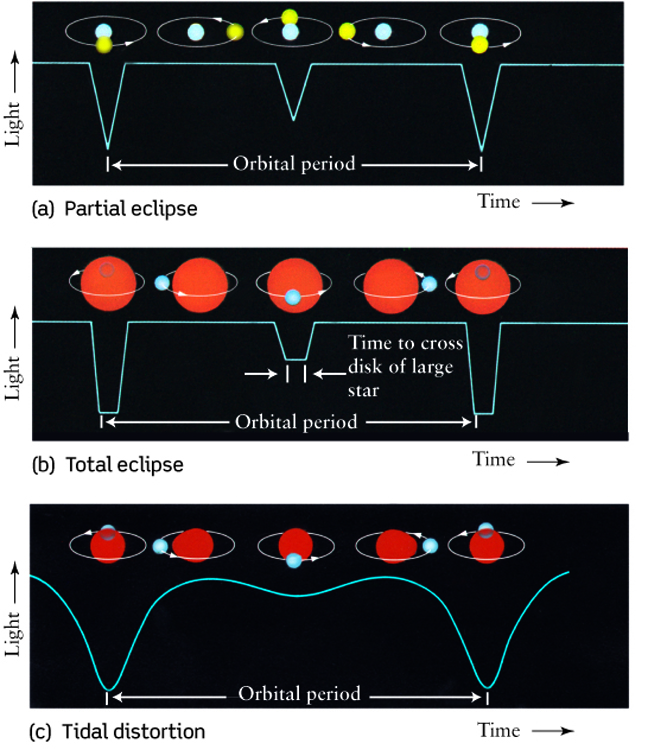Back Next

## Planetary Eclipses

• Another interesting example is one of the new planets found orbiting another star (HD 209458).
• In this case eclipses have been detected.
• When the eclipse occurs, light from the star passes through the planet's atmosphere. The spectral lines which suddenly appear during the eclipse give us the chemical composition of the planet's atmosphere.
• The planet's size is similar to Jupiter.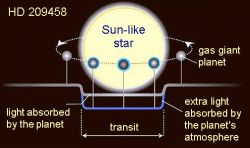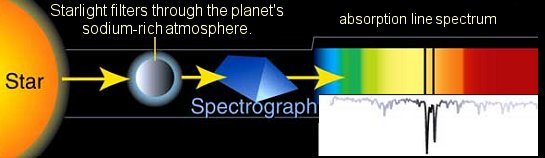Back Next

## Using Binaries to Weigh Stars

• Binary star systems are important because they allow us to find the masses of stars.
• Newton's laws of motion (F=ma) allow us to derive Kepler's equation for orbital motion.
• Kepler's equation:
(M1 + M2) x P2 = a3,
where
• M1 + M2 is the sum of the masses of the two stars, units of the Sun's mass
• a = distance between the two stars, measured in AU
• P = time for one full orbit, measured in years
• The orbital period is usually easy to measure.
• If you can find the orbital separation (a), then you can solve for the sum of the masses.
• If you can also see the distances between the stars and the centre of mass you can also use the Centre-of-Mass equation a1M1 = a2M2 to relate the two masses.
• Combining the two equations (Kepler's equation and the COM equation) we can solve for the two stars' masses.
Back Next

## What are the masses of the stars?

• We can find the masses of the stars in binary systems.
• For stars which are members of the main sequence, mass is correlated with surface temperature and luminosity.
• Main Sequence stars: Heavier stars are hotter and more powerful than light-weight stars.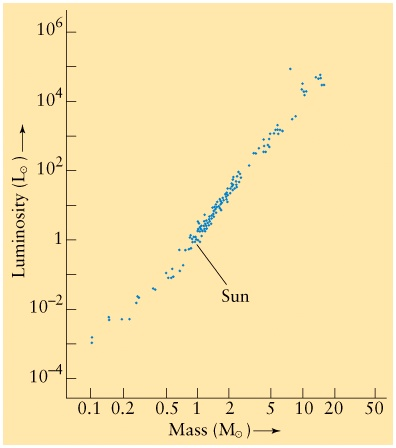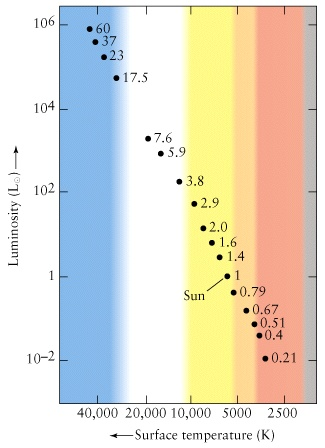Back

Next lecture: Star Formation
Read Chapter 18, pages 471 - 490